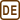## A Hanging Rod

The rod of the length L is hanging motionlessly so that it can rotate around the horizontal axis passing through the top end of the rod. What speed do we have to supply the free end of the rod with so that it stops in the horizontal position?

• #### The moment of inertia

The moment of inertia for the rotation around one of the ends of the homogenous rod of the length L, mass m is according to the table

$I=\frac{1}{3}mL^2.$
• #### Hint

You can use the law of conservation of mechanical energy to solve the problem. Choose two situations in which you will compare the mechanical energy of the rod. Don’t forget to choose the zero level of the potential energy.

It is necessary to supply the end of the rod with speed $v=\sqrt{3gL}$ .